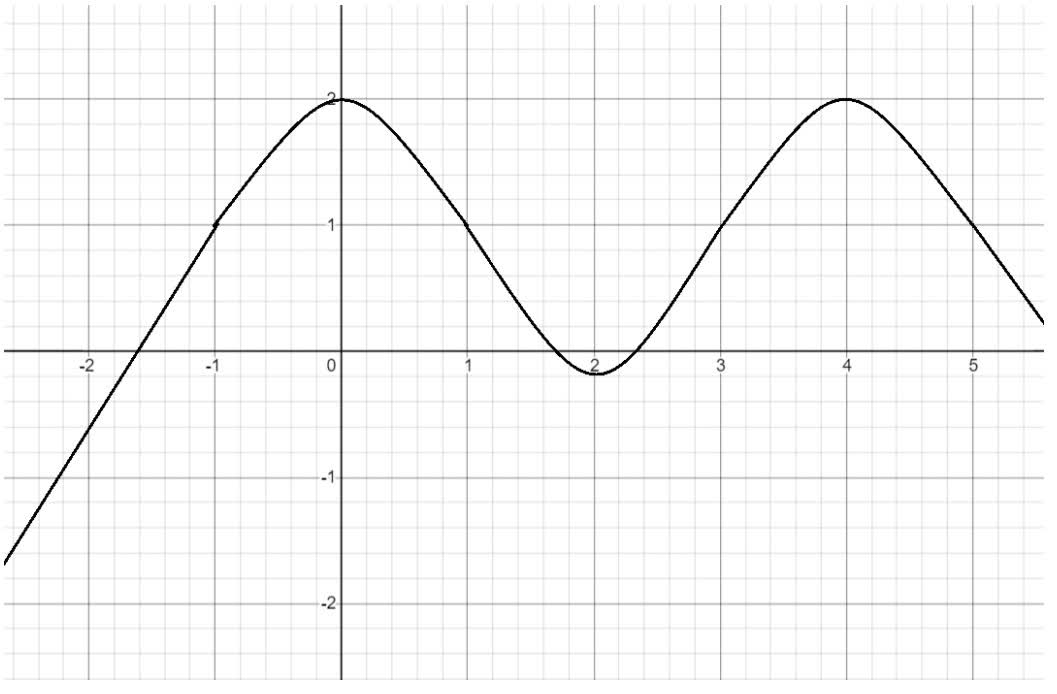Sketch the graph of a function that satisfies all of the given conditions.f'(0)=deliredejoker7m 2021-11-20 Answered
Sketch the graph of a function that satisfies all of the given conditions.f'(0)=f'(2)=f'(4)=0, f'(x)>0 if x<0 or 2<x<4, f'(x)<0 if 0<x<2 or x>4, f''(x)>0 if 13

• Questions are typically answered in as fast as 30 minutes

Solve your problem for the price of one coffee

• Math expert for every subject
• Pay only if we can solve itFesion

f is increasing when x<0 and 2 f is decreasing when 04.
f has local minimum at x=2 and local maximum at x=0 and x=4 because of the way it shifts between increasing and decreasing.
f is concave up when 13.
It has inflection points at x=1 and x=3.Result:
f has the local maximum values at x=0 and x=4, and it has a local minimum value at x=2.